### Home > CALC > Chapter Ch2 > Lesson 2.3.1 > Problem2-108

2-108.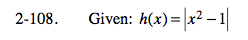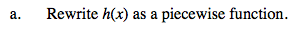Notice how this y = |x² − 1| graph differs from y = x² − 1. From this we can determine that there will be three pieces, and we already know the first and last piece.

The middle piece is a vertical reflection of y = x² − 1.

x² − 1, x < −1
y = −x² + 1, −1 ≤ x ≤ 1
x² − 1, x > 1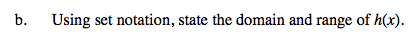Consider how the range of h(x) differs from the range of y = x² − 1.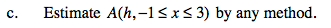A trapezoidal sum is an option.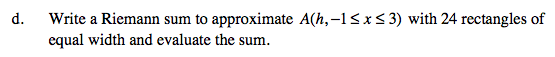The height is determined by the function.

Use the eTool below to help answer each part:
Click on the link to the right to view the full version of the eTool. Calc 2-108 HW eTool